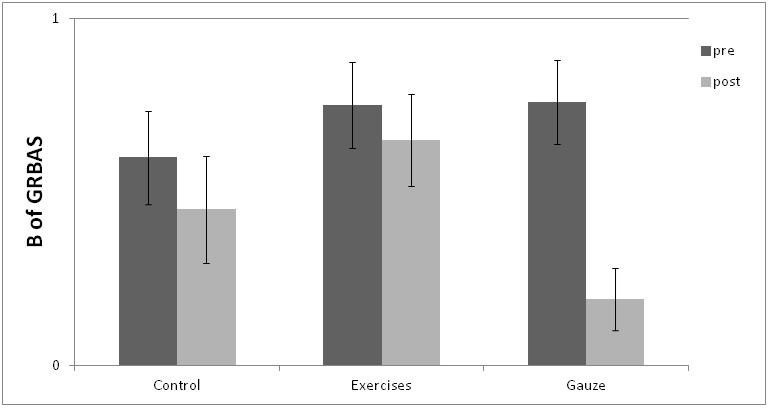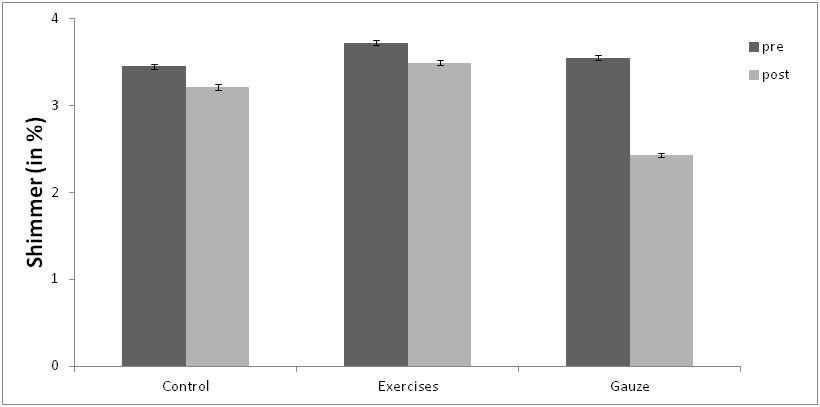# Results (II)

The Amplitude of the mucosal wave showed a significant effect of Test Moment (F(1,58)=30.5, p<.001, η2p=.067) suggesting that the three groups had a greater amplitude in the Post-Test, but with no effect on Treatment Groups (F(2,58)=0.410, p=.665, η2p=.011). The interaction between the two variables was significant (F(2,58)=11.5, p<.001, η2p=.050). To follow up the interaction, as for the other variables, a one-way ANOVA for Pre and Post-Test conditions was carried out. Results showed that in the Pre-Test, mean amplitudes were not significantly different (F(2,38)=1.40, p=.259, η2p=.031), but in the Post-Test condition the difference was significant (F(2,38)=3.67, p=.036, η2p=.098). The unpaired t-test showed that the Gauze group significantly differed from the other groups (Control group: t(19)=2.12, p=.047, Cohen’s d= 0.476 and Exercise group: t(19)=2.35, p=.020, Cohen’s d= 0.567), and no significant differences were found between these two groups (t(19)=0.29, p=.772, Cohen’s d= 0.066), suggesting that the Gauze group showed significantly greater amplitude of mucosal wave after the warmup (see figure 2). The G of GRBAS did not show any significant effect (all p > .12), neither the R (all p > .31), the A (all p > .11) and the S (all p > .13). However, the B showed a significant effect of Test Moment (F(1,58)=15.79, p<.001, 2p=.0565) and interaction between the two variables (F(2,58)=3.20, p=.048, η2p=.026). The Pre-Test one-way ANOVA showed that the three groups were not significantly different (F(2,38)=0.658, p=.524, η2p=.022), but the Post-Test one-way ANOVA was significant (F(2,38)=4.01, p=.027, η2p=.062). The unpaired sample t-test showed that the Gauze group was significantly different from the other two (Control group: t(19)=2.17, p=.043, Cohen’s d= 0.486 and Exercise group: t(19)=3.48, p=.002, Cohen’s d= 0.780) and that the Control and the Exercise groups did not differ significantly (t(19)=0.76, p=.453, Cohen’s d= 0.171), suggesting that only the Gauze group improved breathiness after warm-up (see Figure 3).Figure 3. Bar plots of the B of GRBAS for the Pre- and Post-Test Condition of the three treatments (Control group, Exercise group and Gauze group). Error bars refer to the standard error (SE) of each mean.

Acoustic analysis showed that the F0 variations were not significant (all p > .63) nor the Jitter variations (all p > .53). However, the Shimmer showed a significant effect of Test Moment (F(1,58)=20.55, p<.001, η2p=.079) and Treatment Groups (F(2,58)=3.69, p=.031, η2p=.073) with a significant interaction between the two variables (F(2,58)=6.48, p=.003, η2p=.050), suggesting that the Gauze group was different from the other two.
The pre-test one-way ANOVA analysis showed that the three groups were not significantly different before the treatment (F(2,38)=0.35, p=.703, η2p=.026), but after the treatment they differed significantly (F(2,38)=15.41, p<.001, η2p=.018). The unpaired t-test showed that the Gauze group differed from the other two groups after the treatment (Control group: t(19)=3.31, p=.004, Cohen’s d= 0.741 and Exercise group: t(19)=5.52, p<.001, Cohen’s d= 1.235), while no significant differences were differences were found between Exercise and Control group (t(19)=0.97, p=.341, Cohen’s d= 0.218), suggesting that after the Gauze treatment task participants had significantly better Shimmer values (see Figure 4).Figure 4. Bar plots of the Shimmer (%) for the Pre and Post-Test condition of the three groups (Control group, Exercise group and Gauze group). Error bars refer to the standard error (SE) of each mean.